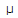# Electronics - Inductors - Discussion

### Discussion :: Inductors - General Questions (Q.No.32)

32.

A 24 V peak source is connected across a 900H inductor. If the frequency of the source is 22 kHz, what is the reactive power?

 [A]. 2.3 VAR [B]. 4.6 VAR [C]. 9.3 VAR [D]. 0 VAR

Explanation:

No answer description available for this question.

 Clara said: (Jan 2, 2012) Reactive Power Q= E^2/XL where XL =2*Pi*f*l ==> 2*3.14*22K*900U ==>124.4 Q = (24)^2/(124.4) Q=4.63 VAR

 Manasa said: (May 16, 2012) Given peak or max value.. Since this is ac ckt, rms must be considered.. Erms=24/square root of 2 R=0,implies power factor=0,implies phase angle phi=90 degree Reactive power=E.I.sin phi E,I are rms values I=E/(2*3.14*22k*900u)

 Rashmi Byakod said: (Jun 21, 2012) Q= Erms^2/XL. Erms=24/square root of 2 ==> 16.97. where XL =2*Pi*f*l ==> 2*3.14*22K*900U ==>124.4. Q = (16.97)^2/(124.4). Q = 2.3VAR.

 Mitesh said: (Aug 23, 2012) XL=2*PI*F*L XL=2*3.14*22K*900u XL=124.4 P=V^2/R P=24^2/124.4 P=4.63VAR BUT HERE 24V PEAK VOLTAGE SOURCE SO P reactive power=P/2=4.63/2 P=2.3VAR

 Nisar said: (Jan 16, 2016) @Clara you missed conversion of Vm to Vrms.

 Deepak said: (May 1, 2018) Reactive Power Q= (E^2)/XL. where XL =2*π*f*l ==> 2*(3.14)*(22000)*(900*(10^(-6))) ==>124.407 ohm. E=Vp*0.707 = 24*0.707 = 16.698 volts, Therefor, Q = ((16.698)^2)/(124.407), Q=2.314 VAR, Reactive power approx 2.3 VAR as per option A.